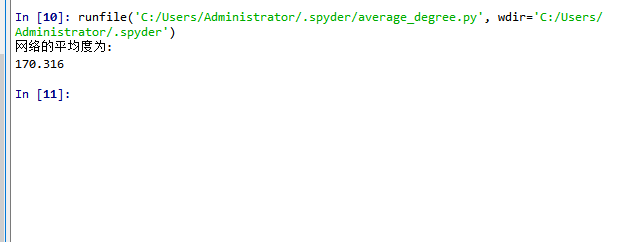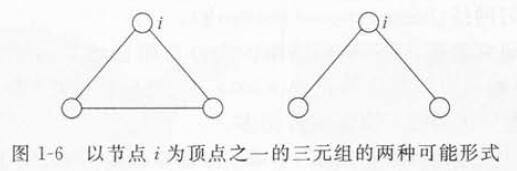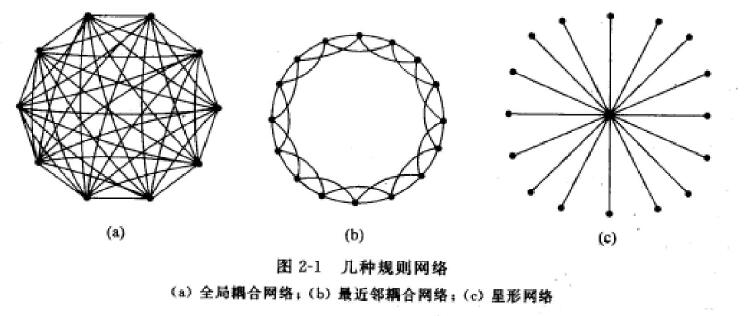• #include "cmath" #include "iostream" #include "fstream" #include "vector" #include "ctime" #include "...#define m 2.5 //平均度&
#include "cmath"
#include "iostream"
#include "fstream"
#include "vector"
#include "ctime"
#include "iomanip"
using namespace std;
using std::vector;

#define N 10000//节点数
#define m 2.5 //平均度<k>=2m
double gamma=3;//gamma值在这改
void fast_search(int a,int b,double A[],double target,int& location){//from large to small
if(a==b){
if(target==A[a])
location=a;
}
if(a>b){
if(A[b]<=target){
location=b;
}
else{
location=0;
}
}
if(a==b-1){
if(A[a]<=target && A[b]>=target){
location=a;
}
if(A[b]<target){
location=b;
}
}
if(a<b-1){
int mid=(a+b)/2;
if(mid==a){
fast_search(mid+1,b,A,target,location);
}
if(mid>a){
if(A[mid]<target){
fast_search(mid,b,A,target,location);
}
if(A[mid]>target){
fast_search(a,mid,A,target,location);
}
if(A[mid]==target){
location=mid;
}
}
}
}

void gui_yi(double A[],int a,int b){
int i;double temp=0;
for(i=a;i<b;i++){
temp+=A[i];
}
for(i=a;i<b;i++){
A[i]=A[i]/temp;
}
}

int v_insert(vector<int>& v,int target){
int i=0;
if(v==-1){
v.insert(v.begin(),target);
return 1;
}
if(v!=-1){
if(v>target){
v.insert(v.begin(),target);
return 1;
}
else if(v==target)
return 0;
else{
while(v[i]!=-1 && v[i]<target)
i++;
if(v[i]==target)
return 0;
else{
v.insert(v.begin()+i,target);
return 1;
}
}
}
}
int lar_rand(){
int a,b,c;
a=rand()%1024;
b=rand()%1024;
c=1024*a+b;
return c;
}
int main(){

vector<int> A[N];
double p[N]={0},x[N]={0},p1,p2,r1,r2,n1024_2=1024*1024,sum;
int i,j,l1=0,l2=0,total_edges=0,return1,return2,i_deg[N]={0},deg={0};

ifstream fin;
ofstream fout;

for(i=1;i<N;i++){
A[i].push_back(-1);
}
for(i=1;i<N;i++)
p[i]=pow((double)i,-(double)(1/(gamma-1)));

gui_yi(p,1,N-1);
sum=0;
for(i=1;i<N;i++){
sum+=p[i];
p[i]=sum;
}

double p_m=p,f_i_j;
double p_m_2=pow((double)1200,2.0);

while(total_edges<m*(N-1)+1){
l1=0;l2=0;
r1=(double)lar_rand()/n1024_2;
r2=(double)lar_rand()/n1024_2;
fast_search(1,N-1,p,r1,l1);
fast_search(1,N-1,p,r2,l2);
if(l1!=0 && l2!=0 && l1!=l2){
return1=v_insert(A[l1],l2);
v_insert(A[l2],l1);
if(return1==1)
total_edges++;
}
}

fout.open("SF_net.txt");
int q;
int k;
int f;
int s=0;
double h=1;
fout<<N<<" "<<0<<endl;
for(i=1;i<N;i++)
{
q=0;
k=0;
f=0;
while(A[i][k]!=-1)
{
k++;
q++;
}
s=s+q;
fout<<i<<" "<<q;
while(A[i][f]!=-1)
{

fout<<" "<<A[i][f]<<" "<<setiosflags(ios::fixed)<<setprecision(6)<<h;
f++;
}
fout<<endl;

}

}    


展开全文• 下面的程序是用来计算网络节点的平均值 # -*- coding: utf-8 -*- """ Created on Sat Nov 30 17:02:21 2019 ...然后除以节点的总数就可以计算出网络节点的平均度 """ def averag...
下面的程序是用来计算网络节点的平均度
# -*- coding: utf-8 -*-
"""
Created on Sat Nov 30 17:02:21 2019

"""

"""

这个程序是来求整个网络的平均度

求解思想，统计出整个网络里面的1的总的个数，
然后除以节点的总数就可以计算出网络节点的平均度

"""

def average_degree():

filestr = ""

with open("result.txt") as files:
for line in files:
filestr += line.strip()

#将字符串转换成列表
matrix = eval(filestr)

nodes = len(matrix)

#存储网络中1的个数，即度的总数
sum_degree = 0

for i in range(len(matrix)):
for j in range(len(matrix)):
if(matrix[i][j] == 1):
sum_degree = sum_degree + 1

print("网络的平均度为:")
aver_degree = float(sum_degree)/nodes
print(aver_degree)

average_degree()展开全文python
• ## 复杂网络

千次阅读 2018-10-28 16:31:28
文章目录复杂网络复杂网络基本概念平均路径长度聚类系数分布网络拓扑基本模型及其性质规则网络全局耦合网络最近邻耦合网络星型耦合网络随机图（ER随机图）小世界网络模型WS小世界模型NW小世界模型小世界网络的...
文章目录复杂网络复杂网络基本概念平均路径长度聚类系数度与度分布网络拓扑基本模型及其性质规则网络全局耦合网络最近邻耦合网络星型耦合网络随机图（ER随机图）小世界网络模型WS小世界模型NW小世界模型小世界网络的小波分析无标度网络模型（重点）基本性质鲁棒性与脆弱性适应度模型
复杂网络
复杂网络基本概念
平均路径长度
两个点$i$和$j$之间的距离$d_{ij}$定义为连接这两个节点的最短路径上的边数。
网络的平均路径长度$L$定义为任意两个节点之间的距离的平均值，即：
$L=\frac{1}{\frac{1}{2}N(N+1)}\sum_{i\ge j}{d_{ij}}$
其中，$N$为网络节点数。
一个含有$N$个节点和$M$条边的网络的平均路径长度可以用时间量级为$O(MN)$的广度优先搜索算法来确定。
网络的平均路径长度也称为网络的特征路径长度。
聚类系数
在你的朋友关系网络中，你的两个朋友很可能彼此也是朋友，这种属性称之为网络的聚类特性。
在网络中的节点$i$有$k_{i}$条边和其他节点连接,即有$k_{i}$个邻居节点。在这$k_{i}$个邻居节点之间最多可能有 $k_{i}(k_{i}-1)/2$条边，而这$k_{i}$个节点之间实际存在的边数$E_{i}$和总的可能的边数 $k_{i}(k_{i}-1)/2$之比就定义为节点$i$的聚类系数$C_{i}$，即：
$C_{i}=\frac{2E_{i}}{k_{i}(k_{i}-1)}$
简单描述即是：
$C_{i}=\frac{与点i相连的三角形的数量}{与点i相连的三元组的数量}$
三元组包含三角形，三元组两种形式为：整个网络的聚类系数$C$就是所有结点i的聚类系数$C_{i}$的平均值。
度与度分布
度是单独节点的属性中简单而又重要的概念，分为出度和入度。直观上，一个节点的度越大，那么这个节点在某种意义下越重要。网络中的所有节点$i$的度$k_{i}$的平均值成为网络的(节点)平均度，记为$\langle k \rangle$，网络中节点的度的分布情况用分布函数$P(k)$来描述。$P(k)$表示一个随机选定的节点的度是$k$的概率。

规则图为Delta分布
随机网络和小世界网络为近似Poisson分布
指数分布（暂未知是哪种网络！）
无标度分布，即幂律分布

无标度分布即是分布函数$f(x)$满足无标度条件：
$f(ax)=bf(x)$
当满足这个条件是必定有：(这里假设     $f(1)f'(1)\neq 0$  )
$f(x) = f(1)x^{-r}, r = -\frac{f(1)}{f'(1)}$
证明见《复杂网络理论及其应用》中P12。
幂指数一般为$2\le r\le 3$，绝大多数节点的度相对很低，只有少量节点的度相对很高，因此这类网络为非均匀网络，那些度相对很高的节点成为网络的集线器（hubs）。
网络拓扑基本模型及其性质
规则网络
全局耦合网络
构成：任意两个点之间都直接相连接，如下图a所示
平均路径长度：1
最大聚类系数：1
最近邻耦合网络
构成：每个节点只和它周围的邻居节点相连，一般具有周期边界条件的最近邻耦合网络包含N个围成一个环的点，如下图b所示，每个节点与它最近的4个节点相连
K：最近邻的个数，图b中为4
N：节点的数目
聚类系数为：
$C_{nc}=\frac{3(K-2)}{4(K-1)}\approx \frac{3}{4}$
平均路径长度为：
$L_{nc} \approx \frac{N}{2K} \rightarrow \infty \quad \quad （N \rightarrow \infty ）$
星型耦合网络
构成：一个点为中心点，其他点与这个中心点直接连接，如下图c所示
聚类系数为：
$C_{star}=\frac{N-1}{N} \rightarrow 1 \quad \quad （N \rightarrow \infty ）$
平均路径长度为：
$L_{star}=2- \frac{2(N-1)}{N(N-1)} \rightarrow 2 \quad \quad （N \rightarrow \infty ）$总体而言，这三种规则网络在很理想的情况下进行建模，很大程度上无法反映真实网络世界情况，是复杂网络研究中最基本的模型，不过有必要对他们的三要素进行理解与学习。
在人工构建的网络中，P2P为完全耦合网络，C/S为星型网络模型，路网在一定程度上可以认为是最近邻耦合模型（这个是自己想的）。
随机图（ER随机图）
构成：假设有大量的纽扣（$N \gg 1$）散落在地上，并以相同的概率$p$给每对纽扣系上一根线，这样便可以得到一个有$N$个点，约$pN(N-1)/2$条边的ER随机图实例。
性质存在的定义：如果当$N \rightarrow \infty$时产生了一个具有性质Q的ER随机图的概率为1，那么就称几乎每一个ER随即图都具有性质Q。
ER随即图的许多性质都是突然涌现的。比如当概率$p$大于某个临界值$p_{c} \propto (lnN)/N$，那么几乎每一个图都是连通的。
平均度：$\langle k \rangle=p(N-1) \approx pN$
平均路径长度：$L_{ER} \propto lnN/ln\langle k \rangle$。在ER图中随机选择一个点，网络中大概有$\langle k \rangle^{L_{ER}}$个其他的点与该点之间的距离等于或者非常接近于$L_{ER}$，因此$N\propto \langle k \rangle^{L_{ER}}$，变换一下形式即得原式。这种平均路径长度为网络规模的对数增长函数的特性就是典型的小世界特征。
聚类系数：$C=p=\langle k \rangle/N\ll1$，这意味着大规模的稀疏ER随机图没有聚类特征。
ER图又称为“Poission随机图”，固定ER随机图的平均度$\langle k \rangle$不变，则对于充分大的N，由于每条边的出现与否都是独立的，ER随机图的度分布可以用Poission分布来表示，即：
$P(k)=C_{N}^{k}p^{k}(1-p)^{N-k} \approx \frac {{\lang k \rang}^k e^{- \lang k \rang}}{k!}$
小世界网络模型
考虑到规则的最近邻耦合网络具有高聚类特性，但是平均路径很大，不是小世界网络，而ER随机图有较小的平均路径长度，但是不具备高聚类特性。将这两者的性质结合，即可得小世界网络模型。
WS小世界模型
构造算法：从最近邻耦合网络这个规则网络开始，将它的边以概率$p$进行随机化重连，一个节点固定，另一个节点随机选择。并保证任意两个不同节点之间至多一条边，节点不能有边与自身相连。$p=0$到$p=1$的过程即是从完全规则网络到完全随机网络的过程。
聚类系数：
$C(p)=\frac{3(K-2)}{4(K-1)}(1-p)^3$
平均路径长度（目前暂无精确解析表达式，利用重正化群方法可以得到如下公式）：
$L(p)=\frac{2N}{K}f(NKp/2)$
其中$f(u)$为一普适标度函数，满足：
f(u)= \left \{ \begin{aligned} constant，u \ll 1 \\ (lnu)/u，u \gg 1 \end{aligned} \right.
后来，基于均场方法有如下表达式：
$f(x) \approx \frac{1}{2\sqrt{x^2+2x}}arctanh\sqrt{\frac{x}{x+2}}$
度分布：由于每个节点有$K$个邻居节点，在随机化重连的过程中，对于每个节点而言，有$K/2$条边是不会离开该节点的，因此，当$k<K/2$时，$P(k)=0$，当$k\geq K/2$时，分布如下：
KaTeX parse error: No such environment: equation* at position 8:
\begin{̲e̲q̲u̲a̲t̲i̲o̲n̲*̲}̲
P(k)= \sum_{n=…
WS小世界模型构造方式中的随机化过程可能破坏网络的连通性，于是：
NW小世界模型
构造算法：从最近邻耦合网络这个规则网络开始，将它的边以概率$p$进行随机化加边。并保证任意两个不同节点之间至多一条边，节点不能有边与自身相连。$p=0$对应原始最近邻耦合网络，而$p=1$对应全局耦合网络。
聚类系数：
$C(p)=\frac{3(K-2)}{4(K-1)+4Kp(p+2)}$
平均路径长度（同WS小世界模型）：
$L(p)=\frac{2N}{K}f(NKp/2)$
其中$f(u)$为一普适标度函数，满足：
f(u)= \left \{ \begin{aligned} constant，u \ll 1 \\ (lnu)/u，u \gg 1 \end{aligned} \right.
后来，基于均场方法有如下表达式：
$f(x) \approx \frac{1}{2\sqrt{x^2+2x}}arctanh\sqrt{\frac{x}{x+2}}$
度分布：由于每个节点至少有$K$个邻居节点，因此，当$k<K$时，$P(k)=0$，当$k\geq K$时，分布如下：
KaTeX parse error: No such environment: equation* at position 8:
\begin{̲e̲q̲u̲a̲t̲i̲o̲n̲*̲}̲
P(k)= C_{N}^{k…
当$p$足够小且$N$足够大时，NW小世界网络本质上等同于WS小世界网络。
小世界网络的小波分析
见《复杂网络理论及其应用》中P23。
大概就是利用小波变化，在一个粗化的状态下，观察网络的统计特性，进而研究在粗化状态下，$LL_{1}$低频区间所展现出来的网络特性，即网络的平均路径长度以及聚类系数。
无标度网络模型（重点）
基本性质
ER随机图和WS小世界模型的一个共同特征是度分布近似为Poisson分布，这样度基本上存在于平均度$\langle k \rangle$峰值附近，当$k\gg\langle k \rangle$时，度为$k$的节点几乎不存在。而许多现实生活中的网络，如Internet、WWW、新陈代谢网络等的连接度分布函数具有幂律形式，由于这类网络的节点的连接度没有明显的特征长度（比如平均度$\langle k \rangle$），因此，称之为无标度网络。
最初是的无标度网络模型：BA无标度网络，通过考虑现实情况下，网络的增长特性（每天都有新的节点和边加入）和优先连接特性（新的节点更加倾向于与那些高度节点相连接，“富者更富”“马太效应”）。该部分为我目前的研究重点。
**BA无标度网络构造算法：**从一个具有$m_{0}$个节点的网络开始，每次加入一个节点并连接到$m$个已存在的节点上，当然，必须满足$m \leq m_{0}$。在连接时，一个新的节点与一个已经存在的节点$i$之间的连接概率$\Pi_{i}$与节点$i$的度$k_{i}$和所有其他节点的度关系如下：
$\Pi_{i}=\frac{k_{i}}{\sum\limits_{j}{k_{j}}}$
平均路径长度：
$L \propto \frac{logN}{loglogN}$
$L$与$logN$一个量级，表明该网络具有小世界特性
聚类系数：
$C=\frac{m^2(m+1)^2}{4(m-1)}\big[ln\frac{m+1}{m}-\frac{1}{m+1}\big]\frac{[ln(t)]^2}{t}$
$m$：每次一个新的节点与$m$个已经存在的节点连接
$t$：经过$t$步，即总共加入$t$个节点，最后共有$N=t+m_{0}$个节点，大概$mt$条边
与ER随即图类似，当网络规模充分大时，BA无标度网络不具有明显的聚类特征
度分布：
对于无标度网络的度分布研究主要有三种方法：连续场理论，主方程法，速率方程法。
主方程法的结果：
定义$p(k,t_{i},t)$为在$t_{i}$时刻记录的节点$i$在$t$时刻的度恰好是$k$的概率。在BA模型中，当一个新节点加入到系统中来时，节点$i$的度增加$1$的概率为$m\Pi_{i}=\frac{k}{2t}$ （根据前文，可以推导出来），否则该节点的度保持不变。那么有：
$<Empty \space Math \space Block>$
$p(k,t_{i},t+1)=\frac{k-1}{2t}p(k-1,t_{i},t)+(1-\frac{k}{2t})p(k,t_{i},t)$
网络的度分布为：
$P(k)=\lim_{t\to +\infty}\big(\frac{1}{t}\sum\limits_{t_{i}}p(k,t_{i},t)\big)$
它满足如下递推方程式：
P(k)= \left \{ \begin{aligned} \frac{k-1}{k+2}P(k-1)，k \geq m+1 \\ \frac{2}{m+2} \quad \quad ，k =m \qquad \end{aligned} \right.
从而可得BA网络的度分布函数为：
$P(k)=\frac{2m(m+1)}{k(k+1)(k+2)} \propto 2m^2k^{-3}$
$m$：每次一个新的节点与$m$个已经存在的节点连接
$k$：随机取一个节点，度为$k$的概率$P(k)$
表明：BA无标度网络的度分布函数可以由幂指数为3的幂律函数近似描述
缺陷：BA无标度网络的幂指数固定为3
鲁棒性与脆弱性
见《复杂网络理论及其应用》中P29。
无标度网络对随机故障策略，即随机移除一些点，有很高的鲁棒性；对蓄意攻击策略，即移除网络中部分度最高的节点，表现得非常脆弱。其中表现情况以整个图的连通性为标准。
鲁棒但又脆弱是复杂系统的最重要和最基本的特征之一。
Broder等人研究了更大规模WWW子网络的鲁棒性。他们发现只有删除所有度大于5的节点才能完全破坏WWW的连通性。这其实是因为WWW具有高度倾斜的度分布，度数大于5的节点在整个网络中所占的比例还是很小的。（这个发现以及相关的具体数值的研究，可能对我的研究有帮助）
适应度模型
BA模型只能生成度分布的幂律指数固定为3的无标度网络，而各种实际复杂网络的幂律指数则不甚相同，且大多属于2到3的范围内。
实际网络常常还具有一些非幂律特征，如指数截断，小变量饱和等。
在BA无标度网络的增长过程中，节点的度也在发生变化并且满足如下幂律关系（流式处理中，应该很有用）：
$k_{i}(t)=\big(\frac{t}{t_{i}}\big)^{\frac{1}{2}}$
$k_{i}(t)$：为第$i$个节点在时刻$t$的度
$t_{i}$：为第$i$个节点加入到网络中的时刻
则可以有一个很直观的认识：就是越老的节点具有越高的度，在完全随机的情况下，这一点基本成立。
适应度模型构造算法：在原有的BA模型上，为每个节点增加了一个适应度权值
从一个具有$m_{0}$个节点的网络开始，每次加入一个节点并连接到$m$个已存在的节点上，当然，必须满足$m \leq m_{0}$，每一个节点的适应度按照概率分布$\rho ( \eta )$选取。在连接时，一个新的节点与一个已经存在的节点$i$之间的连接概率$\Pi_{i}$与节点$i$的度$k_{i}$以及适应度$\eta_{i}$和所有其他节点的度关系如下：
$\Pi_{i}=\frac{\eta_{i}k_{i}}{\sum\limits_{j}{\eta_{j}k_{j}}}$


展开全文无标度网络 BA模型
• 复杂网络中心性1. 中心（Degree Centrality）2. 介数中心（Betweenness Centrality）3. 接近中心（Closeness Centrality） 1. 中心（Degree Centrality） 中心（Degree Centrality）是在网络分析中...
复杂网络中心性1. 度中心性（Degree Centrality）2. 介数中心性（Betweenness Centrality）3. 接近中心性（Closeness Centrality）
1. 度中心性（Degree Centrality）
度中心性（Degree Centrality）是在网络分析中刻画节点中心性（Centrality）的最直接度量指标。一个节点的节点度越大就意味着该节点的度中心性越高，该节点在网络中就越重要。
某个节点 度中心性 计算公式如下：
$DC_i=\frac{k_i}{N-1}$
其中：

$k_i$ 表示现有的与节点 $i$ 相连的边的数量
$N-1$ 表示节点 $i$ 与其他节点都相连的边的数量

例：2. 介数中心性（Betweenness Centrality）
节点介数是指一个网络里通过节点的最短路径条数
某个节点的 介数中心性 的计算公式如下：
$BC_i=\sum_{s\neq i\neq t}\frac{n^i_{st}}{g_{st}}$
其中：

$n^i_{st}$ 表示经过节点 $i$ ，且为最短路径的路径数量
$g_{st}$ 表示连接 $s$ 和 $t$ 的最短路径的数量

归一化（令结果 < 1）后，有：
$BC_i=\frac{1}{(N-1)(N-2)/2}\sum_{s\neq i\neq t}\frac{n^i_{st}}{g_{st}}$
例：上图计算节点 $1$ 的介数中心性：

从 $5$ -> $4$ ，最短路径为 $(5,1,4)$， 该路径经过节点 $1$ ，所以 $n^1_{54}=1,g_{54}=1$
从 $5$ -> $3$ ，最短路径为 $(5,3)$， 该路径不经过节点 $1$，所以 $n^1_{53}=0,g_{53}=1$
从 $5$ -> $2$ ，最短路径为 $(5,1,2),(5,3,2)$， 经过节点 $1$ 的路径为 $(5,1,2)$，所以 $n^1_{52}=1,g_{52}=2$
从 $4$ -> $3$ ，最短路径为 $(4,1,2,3),(4,1,5,3)$， 两条路径都经过节点 $1$，所以 $n^1_{43}=2,g_{43}=2$
从 $4$ -> $2$ ，最短路径为 $(4,1,2)$， 该路径经过节点 $1$，所以 $n^1_{42}=1,g_{42}=1$
从 $3$ -> $2$ ，最短路径为 $(3,2)$， 该路径不经过节点 $1$，所以 $n^1_{32}=0,g_{32}=1$
最后得出 $B(1)=\frac{7}{2}$ ，对其归一化得 $B(1)=\frac{7}{12}$

3. 接近中心性（Closeness Centrality）
接近中心性用于衡量节点重要性
某个节点的 接近中心性 $CC_i$ 为：
$d_i=\frac{1}{N-1}\sum^{N}_{j=1}d_{ij} \quad \quad CC_i=\frac{1}{d_i}$
其中 $d_i$ 表示节点 $i$ 到其余各点的平均距离，平均距离的倒数就是接近中心度
例：以上图节点 $A$ 为例，图中点的个数 $N=11$：

与 $A$ 相连的路径为 $1$ 的共 $4$ 个点，为 $D,E,F,B$
与 $A$ 相连的路径为 $2$ 的共 $3$ 个点，为 $G.C,H$
与 $A$ 相连的路径为 $3$ 的共 $3$ 个点，为 $I,J,K$
可得 $A$ 的平均距离为 $d(A)=\frac{1}{10}(4+2 *3+3*3)$，$A$ 的接近中心度为 $CC(A)=\frac{1}{d(A)}$


展开全文• 中心概念说明： 指标名称 概念 比较 实际应用 点中心 在某个点上，有多少条线 强调某点单独的价值 ★作为基本点的描述 接近中心 该点与网络中其他点距离之和的倒数，越大说明越在...r语言 练习
• 2.2.度、平均度、度分布 2.3.路径、距离与介数 2.4.集聚系数 2.5.网络稀疏性与联通性 2.6.度相关性 2.7.富人俱乐部 2.8.有向网络 2.9.加权网络 2.1复杂网络的表达方式 哥尼斯堡七桥问题 1736年 欧拉定理 如果图...
• 包括BA网络构建，邻接矩阵存储，分布计算与存储等，此部分用C++实现，将邻接矩阵和分布分别存储在文件freeScale.txt和文件freeScale_degree.txt最后用python进行可视化操作。 BA网络构建过程参照这篇博客BA无...C++ 邻接矩阵存储
• 此算法在上一篇算法复杂网络——随机网络ER的构造C++实现及测试的基础上用python重写了，在编写过程中发现python对数组的初始化非常方便，仅用np.zeros（）函数就可以生成，而C++要用双层for循环才可以。在这里我...
• ## 复杂网络的研究

千次阅读 2018-11-04 21:53:53
1. 复杂网络定义 ： 复杂网络概念最开始的时候是相对于规则网络和随机网络提出来的，即介于规则网络和随机网络之间的网络都可以称之为复杂网络。—狭义的复杂网络 从广义上说，任何网络都可以称之为复杂网络，...
• ## 复杂网络综述

万次阅读 多人点赞 2016-11-05 09:44:55
本人毕业设计是关于复杂网络的，之前完全没听说过的概念，于是就在网上找了一些论文来看，顺便做下笔记，这篇文章主要讲了复杂网络的一些基础概述。 这里的网络不是（不仅仅是）计算机网络这门课中的网络，它表示的...结构
• 常见的复杂网络分析方法基本分析方法关联分析方法 注：本文部分内容来自《复杂网络分析与应用》与《中国航空复杂网络的结构特征与应用分析》 1.赵正旭,郭阳,等.复杂网络分析与应用[M]北京:科学出版社,2018. 2.陈...
• ## 复杂网络分析总结

万次阅读 多人点赞 2018-04-08 15:31:40
复杂网络的特点2. 社区检测3. 结构平衡4. 影响最大化5. 网络传播6. 补充7. 参考文献　在我们的现实生活中，许多复杂系统都可以建模成一种复杂网络进行分析，比如常见的电力网络、航空网络、交通网络、计算机网络...可视化
• 不同于规则网络和随机网络，复杂网络具有小世界效应和无标度特性。 小世界效应：大的簇系数和小的平均距离。  无标度特性：节点服从幂律分布—具有某个特定的节点数目与这个特定之间的关系可用一个幂函数...
• 复杂网络研究及其前沿概述1 引言2 复杂网络研究史2.1 七桥问题2.2 随机图理论2.3 小世界网络、无标度网络3 复杂网络的研究特点3.1 网络规模大3.2 网络结构具有复杂性和多元性3.3 网络具有时空复杂性3.4 复杂网络存在...
• ## 图与复杂网络

千次阅读 2018-09-24 12:21:14
图与复杂网络 图论 特点： 它们的目的都是从若干可能的安排或方案中寻求某种意义下的最优安排或方案，数学上把这种问题称为最优化或优化（optimization）问题 是它们都易于用图形的形式直观地描述和表达，数学上把...数学建模
• 文章目录小世界网络复杂网络的特性平均路径长度L聚集系数C分布小世界效应规则网络随机网络小世界网络无标度网络python 代码生成小世界网络规则网络 小世界网络 [百度百科]：小世界网络模型是一类具有较短的...
• 无论是实际网络还是对模型网络进行分析，都离不开对网络拓扑统计指标的计算。反映网络结构与动力学特性的统计指标有很多，Costa等的Characterization of Complex Networks: A Survey of measurements一文对此有全面...
• 咳咳，复杂网络建模project，哈工大深圳课程的项目作业。性能
• 摘要：随着近几年关于复杂网络（Complex network）理论及其应用研究的不断深入，已有大量关于复杂网络的文章发表在Science，Nature，RL，NAS等国际一流的刊物上，侧面反映了复杂网络已经成为物理界的一个新兴的研究...计算机图形学
• ## 复杂网络简单理解

万次阅读 多人点赞 2017-11-28 09:42:18
通俗易懂的复杂网络 1 什么是复杂网络 1.1 直观理解 什么是复杂网络？对普通人而言，在媒体上看到复杂网络，首先想到的是互联网，实际上网络已经成为Internet的代名词，确实Internet从只有几个结点的简单的网络，...
• 很好的入门介绍 关于复杂网络（complex network）和社会网络（social network）。 第一次从学术意义上接触这两个词儿还是...已经记不得是谁的presentation里面有一幅很经典的复杂网络的图了（当时学到的东西太多
• 复杂网络与大数据》第二章：复杂网络模型的学习笔记 目录 1动态演化网络 1.1以网络演化的部件划分 1.2以是否考虑权重划分 1.3以演化网络采用的演化机制划分 1.4以演化网络是否动态变化划分 2社区网络 2.1...
• 将从以下五个方面进行展开（参考书籍《基于复杂网络的机器学习方法》）： 1.相关性 2.距离和路径 3.网络结构 4.网络中心性 5.复杂网络度量方法的分类 1.相关性 密度：衡量网络中各个节点间的...
• 度分布网络中所有节点viv_i的度kik_i的平均值称为网络平均度，记为\，即 <k>=1N∑i=1Nki <k>=\frac{1}{N}\sum_{i=1}^{N}k_i 平均路径长度网络的平均路径长度L定义为任意两个节点之间的距离的平均值，即： L=1...
• 复杂网络社区结构发现算法-基于python networkx clique渗透算法 ] 皮皮blog from: http://blog.csdn.net/pipisorry/article/details/54020333 ref: [ Algorithms ] [ Networkx Reference ]*[ NetworkX ...networkx...